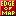# Eye of the Beholder itemtype.dat Format! Wikify formatting

```short int NumberOfItems;
ItemType Items[NumberOfItems];
```
```struct Dice {
unsigned char rolls; // F.e. 4
unsigned char sides; // F.e. T6
unsigned char base;  // F.e. +7
//   =  4T6+7
};
```
```struct ItemType {
unsigned short invbits;    // At which position in inventory it is allowed to be put. See INVENTORY_USAGE_* above.
short int handbits;        // In which hand it can be used
unsigned char profallowed; // Allowed for this profession. See CLASS_USAGE_* above.
unsigned char handallowed; // Allowed for this hand

/* Damage versus small */
Dice damageVsSmall;

/* Damage versus big */
Dice damageVsBig;

char unknown1; // Always 0 in EotB 1
char unknown2; // Always 0 in EotB 2
char unknown3;
};
```
```typedef enum {
INVENTORY_USAGE_NONE     = 0,
INVENTORY_USAGE_QUIVER   = 1 << 0,
INVENTORY_USAGE_ARMOUR   = 1 << 1,
INVENTORY_USAGE_BRACERS  = 1 << 2,
INVENTORY_USAGE_BACKPACK = 1 << 3,
INVENTORY_USAGE_BOOTS    = 1 << 4,
INVENTORY_USAGE_HELMET   = 1 << 5,
INVENTORY_USAGE_NECKLACE = 1 << 6,
INVENTORY_USAGE_BELT     = 1 << 7,
INVENTORY_USAGE_RING     = 1 << 8
} InventoryUsage;
```
```typedef enum {
CLASS_USAGE_NONE    = 0,
CLASS_USAGE_FIGHTER = 1 << 0,
CLASS_USAGE_MAGE    = 1 << 1,
CLASS_USAGE_CLERIC  = 1 << 2,
CLASS_USAGE_THIEF   = 1 << 3,
} ClassUsage;
```